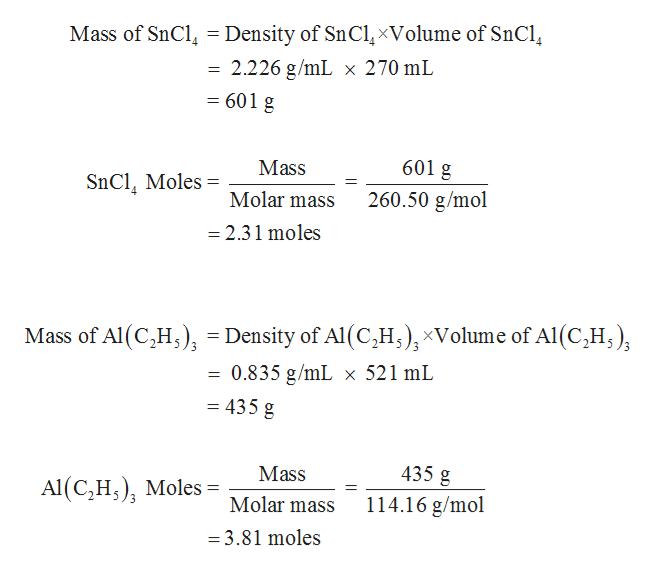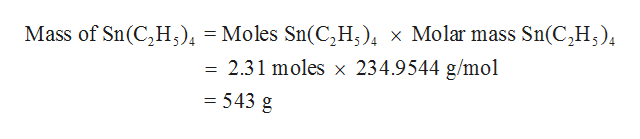# 3SnCl4 + 4Al(C2H5)3  3Sn(C2H5)4 + 4AlCl3In one experiment, 0.270 L of SnCl4 (d = 2.226 g/mL) was treated with 0.521 L of triethylaluminum (Al(C2H5)3); d = 0.835 g/mL).What is the theoretical yield in this experiment (mass of tetraethylstannane, Sn(C2H5)4)? If 0.408 L of tetraethylstannane (d = 1.187 g/mL) were actually isolated in this experiment, what was the percent yield?

Question
91 views

3SnCl4 + 4Al(C2H5)3  3Sn(C2H5)4 + 4AlCl3

In one experiment, 0.270 L of SnCl4 (d = 2.226 g/mL) was treated with 0.521 L of triethylaluminum (Al(C2H5)3); d = 0.835 g/mL).
What is the theoretical yield in this experiment (mass of tetraethylstannane, Sn(C2H5)4)?

If 0.408 L of tetraethylstannane (d = 1.187 g/mL) were actually isolated in this experiment, what was the percent yield?

check_circle

Step 1

The mass and moles of SnCl4 and triethylaluminum is determined as follows,help_outlineImage TranscriptioncloseMass of SnCl = Density of SnClxVolume of SnCl = 2.226 g/mL x 270 mL 601 g 601 g Mass SnCl Moles= 1 Molar mass 260.50 g/mol = 2.31 moles Mass of Al (C,H,, = Density of Al (C,H,)xVolume of Al (C2H, = 0.835 g/mL x 521 mL = 435 g 435 g Mass Al (C,H) Moles 114.16 g/mol Molar mass = 3.81 moles fullscreen
Step 2

Observing the given balanced chemical equation clearly shows that 3 SnCl4 moles reacts with 4 Al(C2H5)3 and forms 3 moles of tetraethylstannane. About 1 mole of SnCl4  requires 4/3 moles of Al(C2H5)3 and  hence, 2.31 moles require...help_outlineImage TranscriptioncloseMass of Sn(C,H,) = Moles Sn(C,H) x Molar mass Sn(C2H) = 2.31 moles x 234.9544 g/mol = 543 g fullscreen

### Want to see the full answer?

See Solution

#### Want to see this answer and more?

Solutions are written by subject experts who are available 24/7. Questions are typically answered within 1 hour.*

See Solution
*Response times may vary by subject and question.
Tagged in
ScienceChemistry

### Inorganic Chemistry